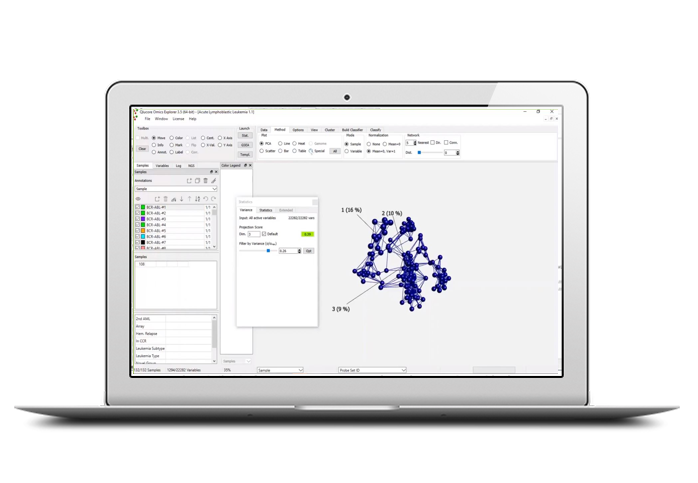# What is the dimension of a data set?

We will describe what the dimension of a data set is and how to know if the first 3 dimensions of the PCA plot is enough to capture the complexity of the data or if we need to go further.
In particular, we will perform Dimension Estimation using the Scree and Talus plots and see what we can learn from them.

Category: Webinar

Short introduction video

Watch here

Get started now with a free 10 days trial of Qlucore Omics Explorer!

Start here